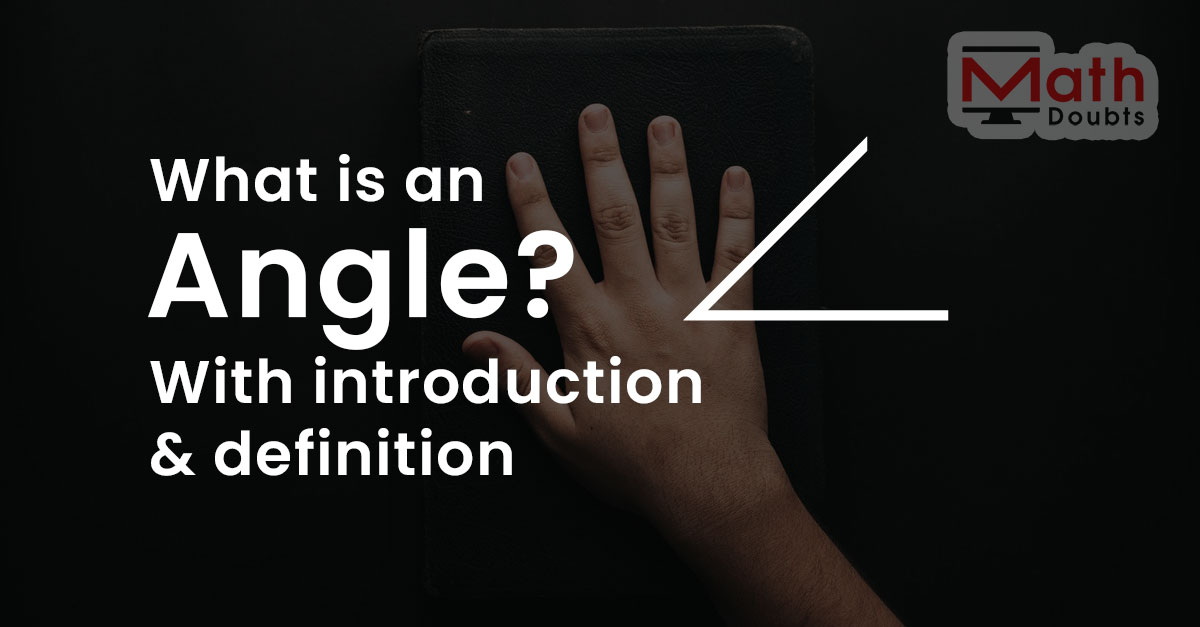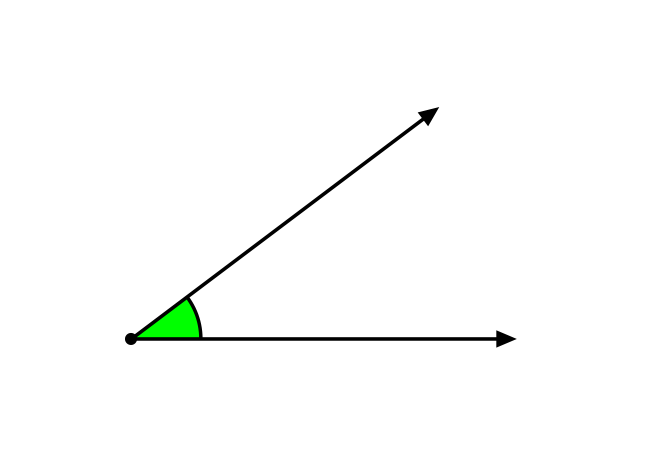# What is an angle?The amount of rotation of a ray from its initial position to final position is called an angle.

## IntroductionAn angle is a basic geometric concept and it is most important for studying the geometric shapes. So, it is essential for everyone to understand what exactly an angle is.

The picture shows the graphical representation of an angle. It is a basic open geometric figure, which is formed by the two non-parallel lines with one common starting point.

Learn at the following two concepts to learn much more about the concept of an angle.

### Representation

According to the geometrical representation of an angle, the symbol $\angle$ is used to represent an angle in mathematics.

### Formation

There is a conflict between two concepts of forming an angle. However, they both explain the concept of an angle geometrically. So, they both are acceptable. Let’s learn both concepts to understand how an angle is formed in geometry.

Latest Math Topics
Jun 26, 2023
Jun 23, 2023

Latest Math Problems
Jul 01, 2023
Jun 25, 2023
###### Math Questions

The math problems with solutions to learn how to solve a problem.

Learn solutions

Practice now

###### Math Videos

The math videos tutorials with visual graphics to learn every concept.

Watch now

###### Subscribe us

Get the latest math updates from the Math Doubts by subscribing us.